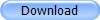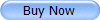FileBack PC 4.1 Fast & Flexible Backup Software for Microsoft Windows
• Home • Product Info • Download • Buy Now! • Quotation • Support • Reference • Help • Forums • Contact MOS •

### <!-- function price(thisform, qty, each) { if (qty > 0) { if ((thisform.version.selectedIndex <= 5) || (thisform.version.selectedIndex >= 12)) { return (Math.ceil(Math.exp(Math.log(0.76)*(Math.log(qty)*2/Math.log(10)))*qty*each)); } else { return (Math.ceil(Math.exp(Math.log(0.80)*(Math.log(qty)*2/Math.log(10)))*qty*each)); } } else { return (0); } } function calctotal(thisform) { q = thisform.quantity.value; qp = thisform.prevquantity.value; thisform.eligibility.value = ""; if (q*1<1) { q=1; thisform.quantity.value = "1"; } prodversion = thisform.version.selectedIndex; if (prodversion > 6) prodversion = prodversion -6; if ((prodversion > 3) && (qp*1 <= 0)) { thisform.prevquantity.value = "1"; qp = 1; } if ((prodversion > 3) && (qp*1 > q*1)) { qp = q; thisform.prevquantity.value = qp; } if (qp*1<0) { qp = 0; thisform.prevquantity.value = "0"; } if (qp*1 == 0) { thisform.eligibility.value = "Not Applicable: New Purchase."; } if ((prodversion < 4) && (thisform.prevdate.selectedIndex == 2) && (qp*1>0)) { thisform.eligibility.value = "Ineligible: Purchase must be less than one year ago."; qp = 0; } if (thisform.version.selectedIndex == 0) { a = price(thisform,q*1+qp*1,55)-price(thisform,qp,55); } else if (thisform.version.selectedIndex == 1) { a = price(thisform,q*1+qp*1,40)-price(thisform,qp,40); } else if (thisform.version.selectedIndex == 2) { a = price(thisform,q*1+qp*1,25)-price(thisform,qp,25); } else if (thisform.version.selectedIndex == 3) { a = price(thisform,q*1+qp*1,15)-price(thisform,qp,15); } else if (thisform.version.selectedIndex == 4) { a = price(thisform,q,45)-price(thisform,qp,35); } else if (thisform.version.selectedIndex == 5) { a = price(thisform,q,45)-price(thisform,qp,25); } else if (thisform.version.selectedIndex == 6) { a = price(thisform,q,35)-price(thisform,qp,25); } else if (thisform.version.selectedIndex == 7) { a = price(thisform,q*1+qp*1,45)-price(thisform,qp,45); } else if (thisform.version.selectedIndex == 8) { a = price(thisform,q*1+qp*1,35)-price(thisform,qp,35); } else if (thisform.version.selectedIndex == 9) { a = price(thisform,q*1+qp*1,25)-price(thisform,qp,25); } else if (thisform.version.selectedIndex == 10) { a = price(thisform,q,45)-price(thisform,qp,35); } else if (thisform.version.selectedIndex == 11) { a = price(thisform,q,45)-price(thisform,qp,25); } else if (thisform.version.selectedIndex == 12) { a = price(thisform,q,35)-price(thisform,qp,25); } else if (thisform.version.selectedIndex == 13) { a = price(thisform,q,55)-price(thisform,qp,20); } else if (thisform.version.selectedIndex == 14) { a = price(thisform,q,55)-price(thisform,qp,15); } else if (thisform.version.selectedIndex == 15) { a = price(thisform,q,40)-price(thisform,qp,15); } else if (thisform.version.selectedIndex == 16) { a = price(thisform,q,55)-price(thisform,qp,10); } else if (thisform.version.selectedIndex == 17) { a = price(thisform,q,40)-price(thisform,qp,10); } else if (thisform.version.selectedIndex == 18) { a = price(thisform,q,25)-price(thisform,qp,10); } thisform.academic.disabled=(q<10); thisform.nonprofit.disabled=(q<10); if ((thisform.nonprofit.checked)&&(thisform.academic.checked)) thisform.academic.checked=false; if ((thisform.academic.checked)&&(q*1>=10)) a = Math.ceil(a*0.75); if ((thisform.nonprofit.checked)&&(q*1>=10)) a = Math.ceil(a*0.80); if (qp*1>0) { if (thisform.prevdate.selectedIndex > 0) { a = a + 5; thisform.eligibility.value = "Eligible, \$5 processing fee applies."; } else if (thisform.prevdate.selectedIndex == 0) { thisform.eligibility.value = "Eligible, no processing fee for first 30 days."; } } if (qp*1>0) { if (thisform.eligibility.value == "") thisform.eligibility.value = "Eligible"; } else { if (thisform.eligibility.value == "") thisform.eligibility.value = "Not Applicable: Not an upgrade."; } thisform.total.value = "\$"+a+".00"; Converter.Amount.value=a+".00"; return(true); } function validate(thisform) { calctotal(thisform); if (thisform.quantity.value*1<10) { alert("Please only use this form for orders of ten or more licenses."); return(false); } else if (thisform.name.value.length<5) { alert("Please enter a your name before attempting to submit your request."); thisform.name.focus(); return(false); } else if (thisform.username.value.length<5) { alert("Please enter a valid e-mail address before attempting to submit your request."); thisform.username.focus(); return(false); } else { return(true); } } // -->FileBack PC Pricing Quotation

Pricing for FileBack PC is determined by a sliding scale, with pricing per license decreasing quickly after quantity one.

For quantities of ten or more licenses, you may lock in the quotation for thirty days.  To do so please fill out the contact portion of the form below and click the "Submit Quotation" button.  Your request will be assigned a unique transaction number, and will be sent to Maximum Output Software and placed on file.  Be sure and print this page before clicking the submit button.

Note: This form requires a JavaScript-enabled browser to function properly.
Do not press Enter to calculate the total; you must click on the "Calculate Total" button.

Product Quotation

Product Version & Edition
License Quantity

Previous License Information (If applicable)
(for determining upgrade pricing and eligibility)
Previous License Quantity leave at "0" for new purchases

Original Purchase Date

Upgrade Status

Applicable Discounts
Academic / Religious Institution (25%)
Nonprofit Organization (20%)

Academic/religious/nonprofit discount only applies to qualifying academic, religious, and nonprofit institutions for orders of quantity ten or greater.   Proof of qualification will  be required.  Previous customer discount applies to new purchases by customers who have purchased other software from MOS other than FileBack PC.  Only one discount per order is permitted.

Quotation Total

Lock in Quotation
(For quantity 10 or greater)
 Your Name Organization E-mail Address Phone Number Please include country code if outside the U.S.A.

When you order you will receive a registration code via e-mail to get you up and running in just a few minutes and free updates to any same major version of the product (i.e. 3.1 to 3.2, or 4.0 to 4.1). The quoted price also includes applicable taxes and shipping and handling.

###All Text, Images, and Scripts © 1997-2019 Maximum Output Software.  All Rights Reserved.